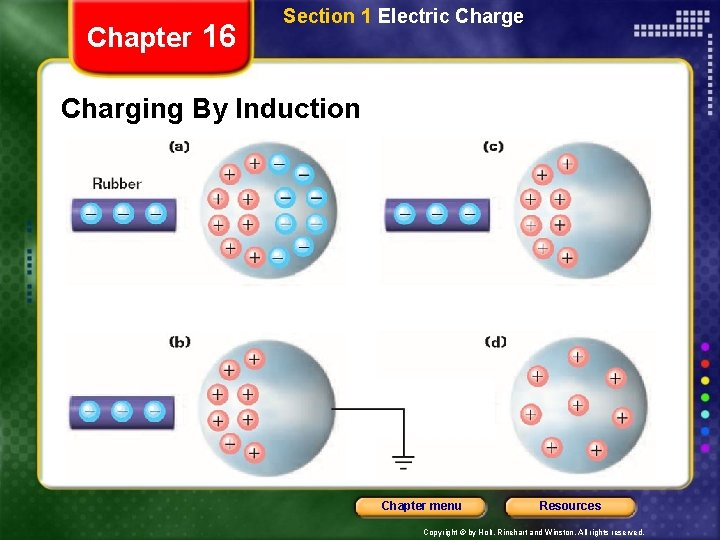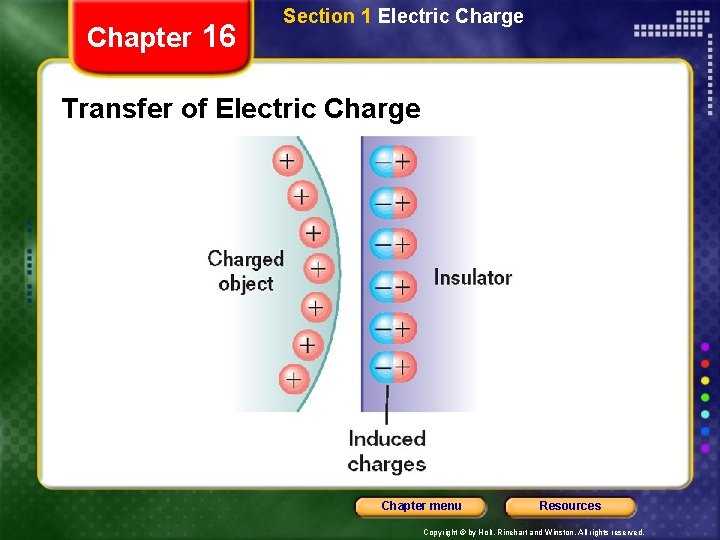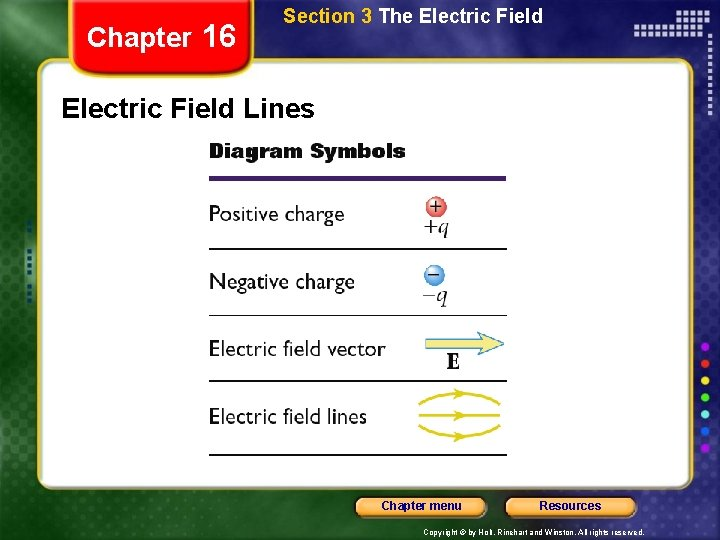Chapter 16 Electric Forces and Fields Table of

• Slides: 83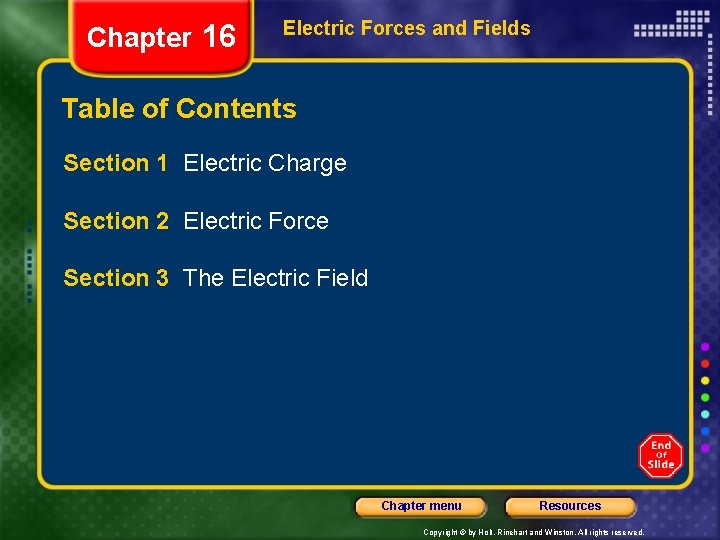Chapter 16 Section 1 Electric Charge Objectives • Understand the basic properties of electric charge. • Differentiate between conductors and insulators. • Distinguish between charging by contact, charging by induction, and charging by polarization. Chapter menu Resources Copyright © by Holt, Rinehart and Winston. All rights reserved.Chapter 16 Section 1 Electric Charge Properties of Electric Charge • There are two kinds of electric charge. – like charges repel – unlike charges attract • Electric charge is conserved. – Positively charged particles are called protons. – Uncharged particles are called neutrons. – Negatively charged particles are called electrons. Chapter menu Resources Copyright © by Holt, Rinehart and Winston. All rights reserved.Chapter 16 Section 1 Electric Charge Properties of Electric Charge, continued • Electric charge is quantized. That is, when an object is charged, its charge is always a multiple of a fundamental unit of charge. • Charge is measured in coulombs (C). • The fundamental unit of charge, e, is the magnitude of the charge of a single electron or proton. e = 1. 602 176 x 10– 19 C Chapter menu Resources Copyright © by Holt, Rinehart and Winston. All rights reserved.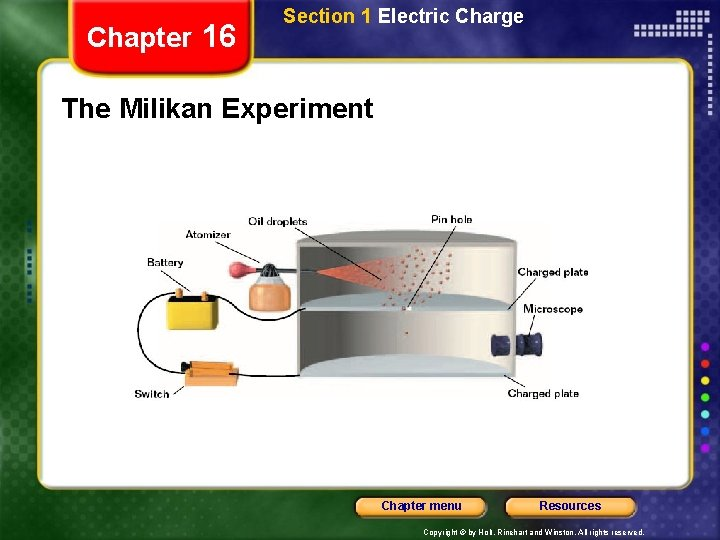Chapter 16 Section 1 Electric Charge Transfer of Electric Charge • An electrical conductor is a material in which charges can move freely. • An electrical insulator is a material in which charges cannot move freely. Chapter menu Resources Copyright © by Holt, Rinehart and Winston. All rights reserved.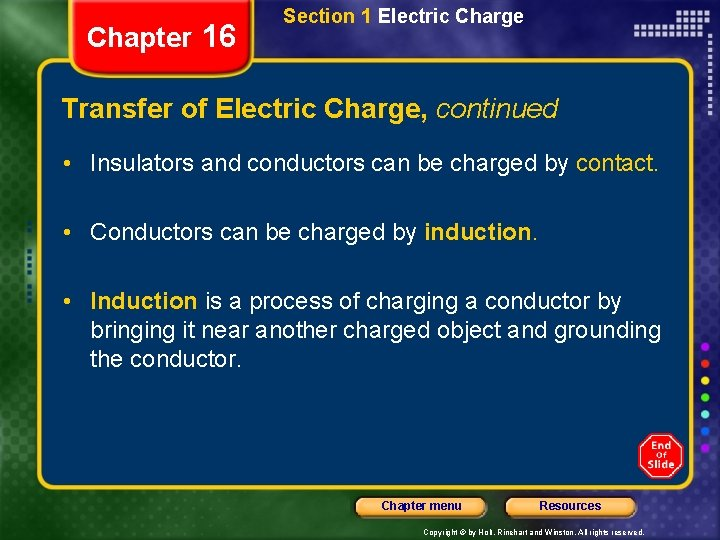Chapter 16 Section 1 Electric Charge Transfer of Electric Charge, continued • Insulators and conductors can be charged by contact. • Conductors can be charged by induction. • Induction is a process of charging a conductor by bringing it near another charged object and grounding the conductor. Chapter menu Resources Copyright © by Holt, Rinehart and Winston. All rights reserved.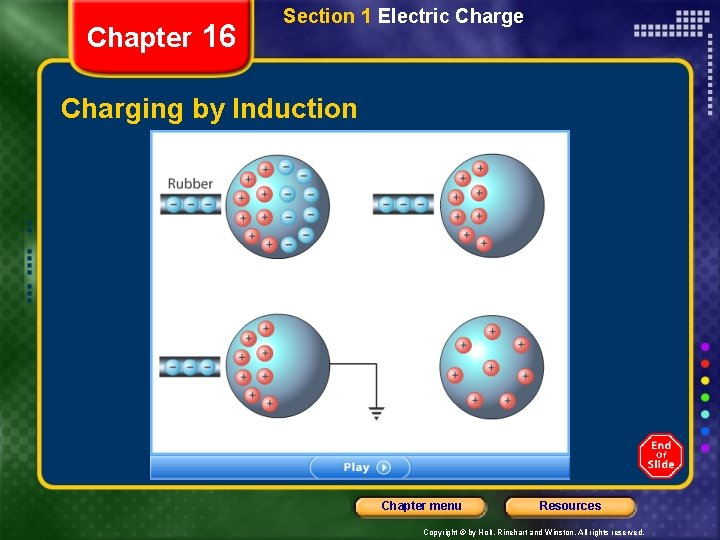Chapter 16 Section 1 Electric Charge Transfer of Electric Charge, continued • A surface charge can be induced on insulators by polarization. • With polarization, the charges within individual molecules are realigned such that the molecule has a slight charge separation. Chapter menu Resources Copyright © by Holt, Rinehart and Winston. All rights reserved.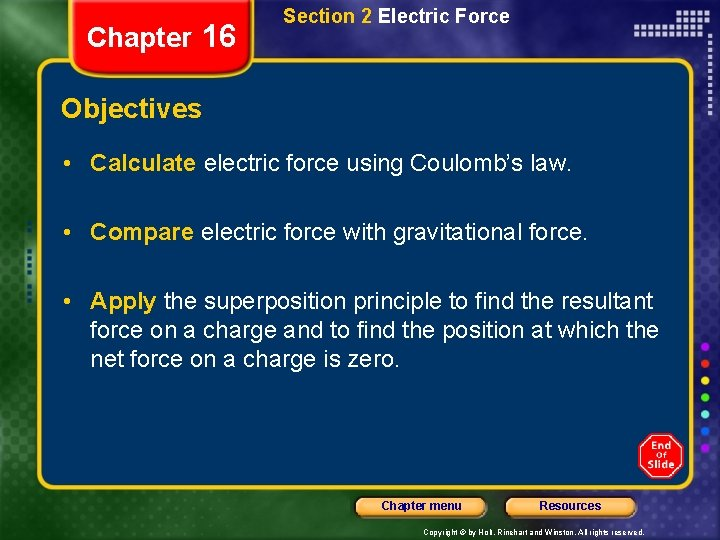Chapter 16 Section 2 Electric Force Objectives • Calculate electric force using Coulomb’s law. • Compare electric force with gravitational force. • Apply the superposition principle to find the resultant force on a charge and to find the position at which the net force on a charge is zero. Chapter menu Resources Copyright © by Holt, Rinehart and Winston. All rights reserved.Chapter 16 Section 2 Electric Force Coulomb’s Law • Two charges near one another exert a force on one another called the electric force. • Coulomb’s law states that the electric force is proportional to the magnitude of each charge and inversely proportional to the square of the distance between them. Chapter menu Resources Copyright © by Holt, Rinehart and Winston. All rights reserved.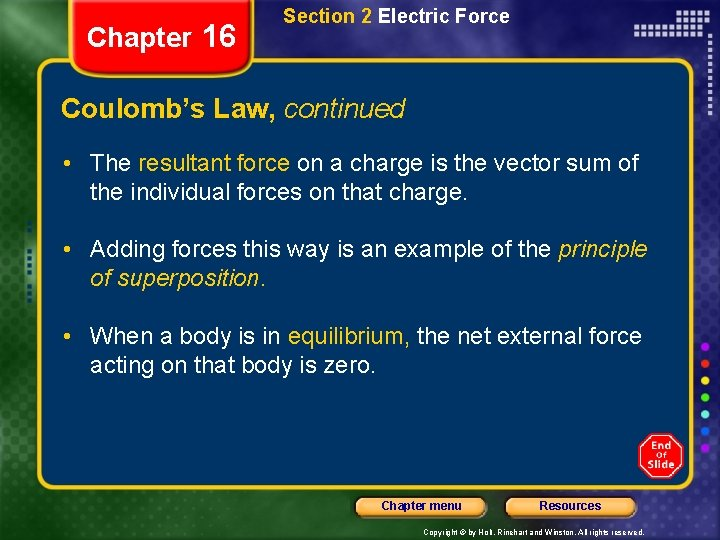Chapter 16 Section 2 Electric Force Coulomb’s Law, continued • The resultant force on a charge is the vector sum of the individual forces on that charge. • Adding forces this way is an example of the principle of superposition. • When a body is in equilibrium, the net external force acting on that body is zero. Chapter menu Resources Copyright © by Holt, Rinehart and Winston. All rights reserved.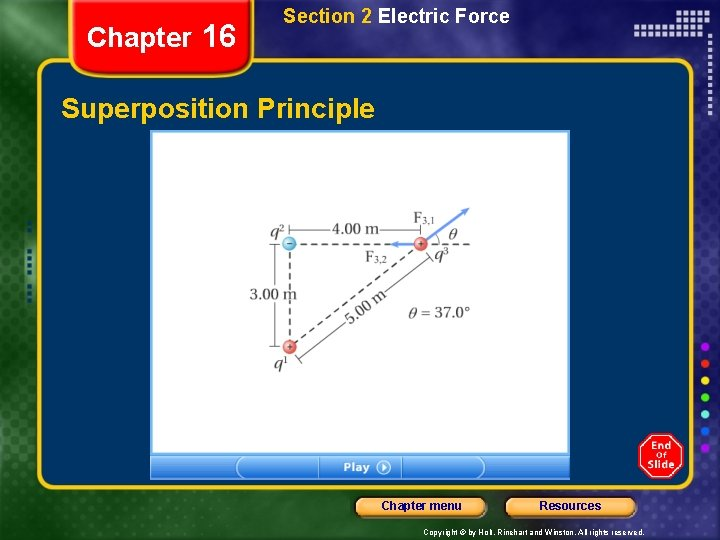Chapter 16 Section 2 Electric Force Sample Problem The Superposition Principle Consider three point charges at the corners of a triangle, as shown at right, where q 1 = 6. 00 10– 9 C, q 2 = – 2. 00 10– 9 C, and q 3 = 5. 00 10– 9 C. Find the magnitude and direction of the resultant force on q 3. Chapter menu Resources Copyright © by Holt, Rinehart and Winston. All rights reserved.Chapter 16 Section 2 Electric Force Sample Problem, continued The Superposition Principle 1. Define the problem, and identify the known variables. Given: q 1 = +6. 00 10– 9 C r 2, 1 = 3. 00 m q 2 = – 2. 00 10– 9 C r 3, 2 = 4. 00 m q 3 = +5. 00 10– 9 C r 3, 1 = 5. 00 m q = 37. 0º Unknown: F 3, tot = ? Diagram: Chapter menu Resources Copyright © by Holt, Rinehart and Winston. All rights reserved.Chapter 16 Section 2 Electric Force Sample Problem, continued The Superposition Principle Tip: According to the superposition principle, the resultant force on the charge q 3 is the vector sum of the forces exerted by q 1 and q 2 on q 3. First, find the force exerted on q 3 by each, and then add these two forces together vectorially to get the resultant force on q 3. 2. Determine the direction of the forces by analyzing the charges. The force F 3, 1 is repulsive because q 1 and q 3 have the same sign. The force F 3, 2 is attractive because q 2 and q 3 have opposite signs. Chapter menu Resources Copyright © by Holt, Rinehart and Winston. All rights reserved.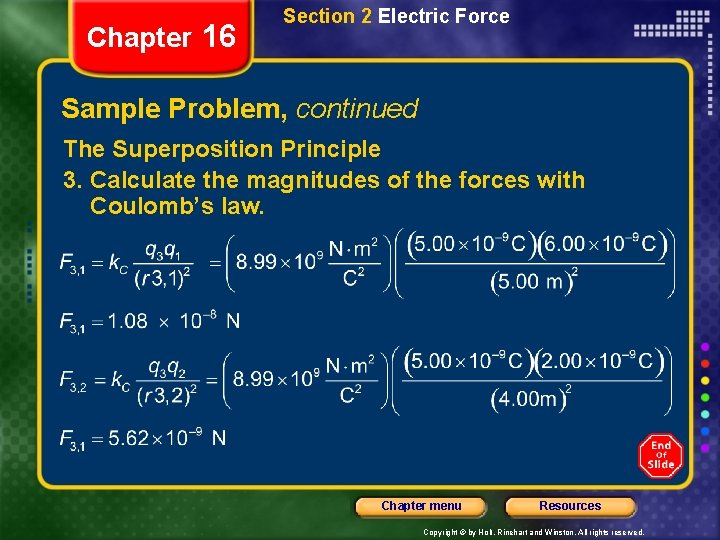Chapter 16 Section 2 Electric Force Sample Problem, continued The Superposition Principle 3. Calculate the magnitudes of the forces with Coulomb’s law. Chapter menu Resources Copyright © by Holt, Rinehart and Winston. All rights reserved.Chapter 16 Section 2 Electric Force Sample Problem, continued The Superposition Principle 4. Find the x and y components of each force. At this point, the direction each component must be taken into account. F 3, 1: Fx = (F 3, 1)(cos 37. 0º) = (1. 08 10– 8 N)(cos 37. 0º) Fx = 8. 63 10– 9 N Fy = (F 3, 1)(sin 37. 0º) = (1. 08 10– 8 N)(sin 37. 0º) Fy = 6. 50 10– 9 N F 3, 2: Fx = –F 3, 2 = – 5. 62 10– 9 N Fy = 0 N Chapter menu Resources Copyright © by Holt, Rinehart and Winston. All rights reserved.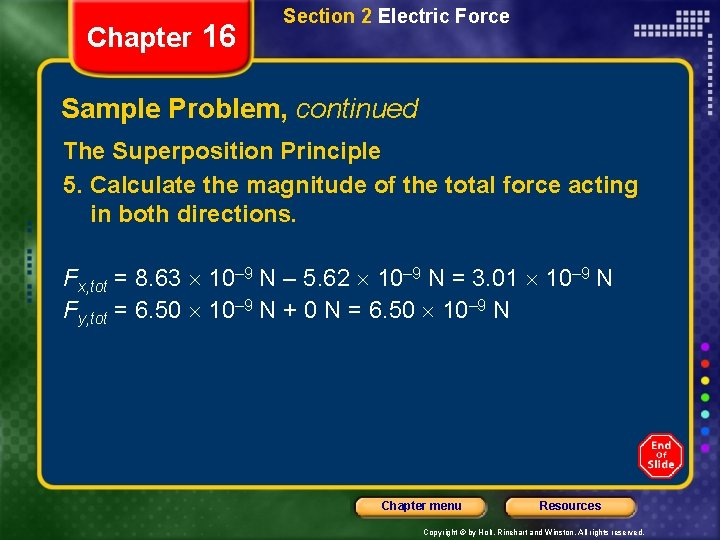Chapter 16 Section 2 Electric Force Sample Problem, continued The Superposition Principle 5. Calculate the magnitude of the total force acting in both directions. Fx, tot = 8. 63 10– 9 N – 5. 62 10– 9 N = 3. 01 10– 9 N Fy, tot = 6. 50 10– 9 N + 0 N = 6. 50 10– 9 N Chapter menu Resources Copyright © by Holt, Rinehart and Winston. All rights reserved.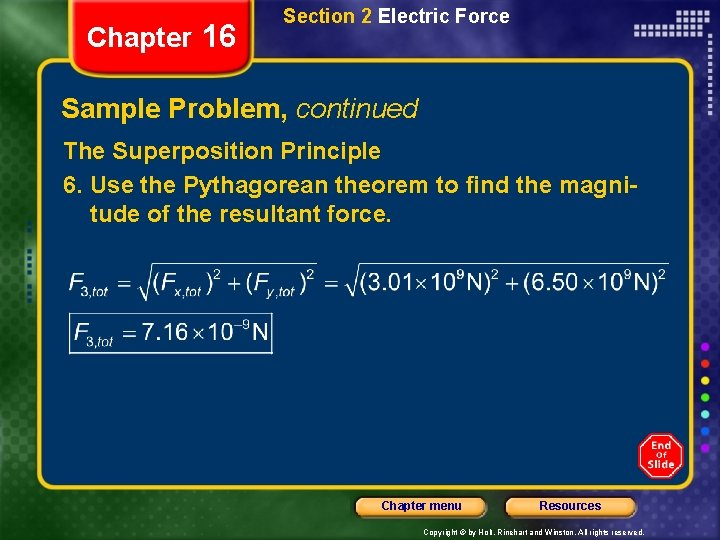Chapter 16 Section 2 Electric Force Sample Problem, continued The Superposition Principle 6. Use the Pythagorean theorem to find the magnitude of the resultant force. Chapter menu Resources Copyright © by Holt, Rinehart and Winston. All rights reserved.Chapter 16 Section 2 Electric Force Sample Problem, continued The Superposition Principle 7. Use a suitable trigonometric function to find the direction of the resultant force. In this case, you can use the inverse tangent function: Chapter menu Resources Copyright © by Holt, Rinehart and Winston. All rights reserved.Chapter 16 Section 2 Electric Force Coulomb’s Law, continued • The Coulomb force is a field force. • A field force is a force that is exerted by one object on another even though there is no physical contact between the two objects. Chapter menu Resources Copyright © by Holt, Rinehart and Winston. All rights reserved.Chapter 16 Section 3 The Electric Field Objectives • Calculate electric field strength. • Draw and interpret electric field lines. • Identify the four properties associated with a conductor in electrostatic equilibrium. Chapter menu Resources Copyright © by Holt, Rinehart and Winston. All rights reserved.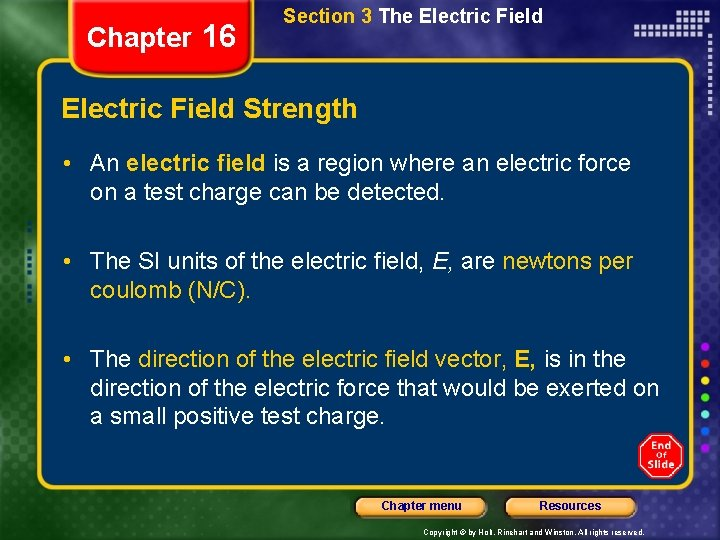Chapter 16 Section 3 The Electric Field Strength • An electric field is a region where an electric force on a test charge can be detected. • The SI units of the electric field, E, are newtons per coulomb (N/C). • The direction of the electric field vector, E, is in the direction of the electric force that would be exerted on a small positive test charge. Chapter menu Resources Copyright © by Holt, Rinehart and Winston. All rights reserved.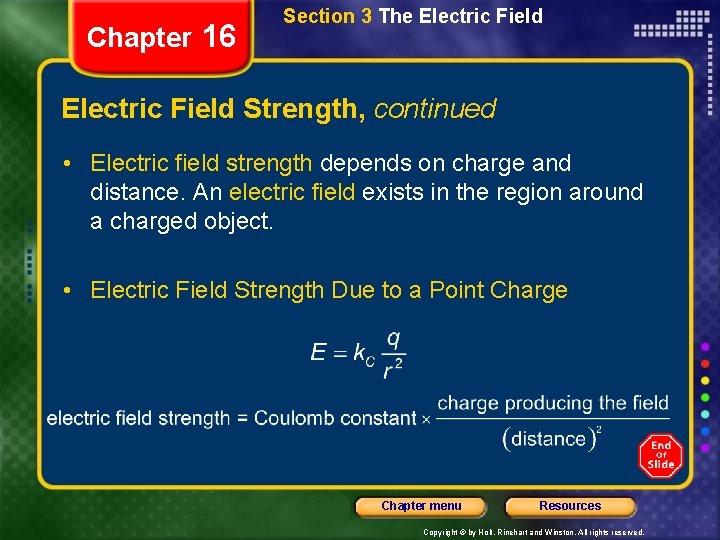Chapter 16 Section 3 The Electric Field Strength, continued • Electric field strength depends on charge and distance. An electric field exists in the region around a charged object. • Electric Field Strength Due to a Point Charge Chapter menu Resources Copyright © by Holt, Rinehart and Winston. All rights reserved.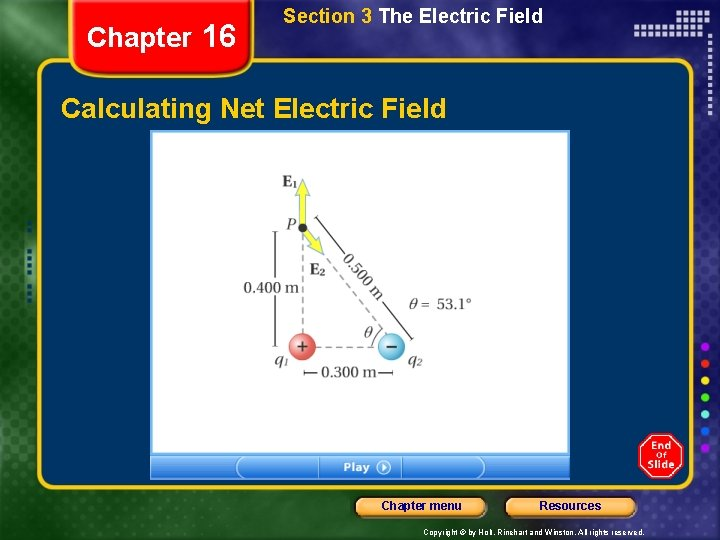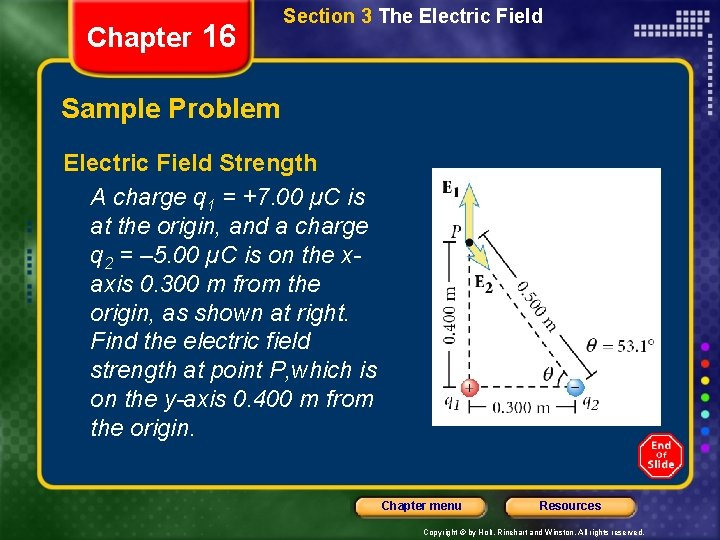Chapter 16 Section 3 The Electric Field Sample Problem Electric Field Strength A charge q 1 = +7. 00 µC is at the origin, and a charge q 2 = – 5. 00 µC is on the xaxis 0. 300 m from the origin, as shown at right. Find the electric field strength at point P, which is on the y-axis 0. 400 m from the origin. Chapter menu Resources Copyright © by Holt, Rinehart and Winston. All rights reserved.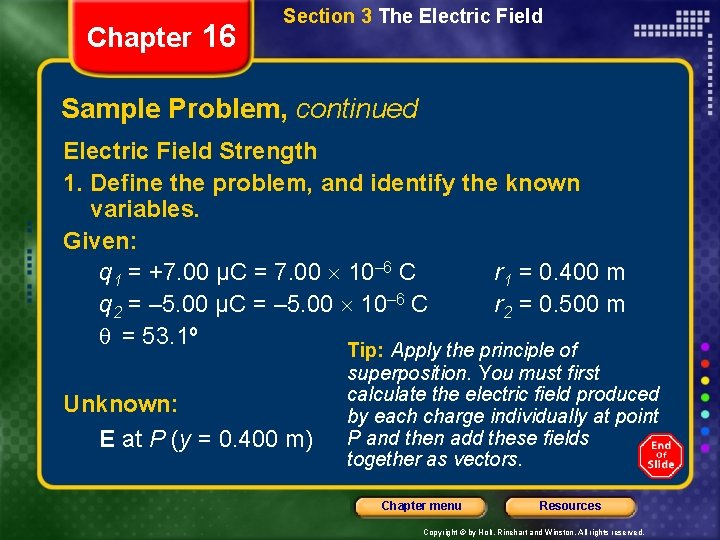Chapter 16 Section 3 The Electric Field Sample Problem, continued Electric Field Strength 1. Define the problem, and identify the known variables. Given: q 1 = +7. 00 µC = 7. 00 10– 6 C r 1 = 0. 400 m q 2 = – 5. 00 µC = – 5. 00 10– 6 C r 2 = 0. 500 m q = 53. 1º Unknown: E at P (y = 0. 400 m) Tip: Apply the principle of superposition. You must first calculate the electric field produced by each charge individually at point P and then add these fields together as vectors. Chapter menu Resources Copyright © by Holt, Rinehart and Winston. All rights reserved.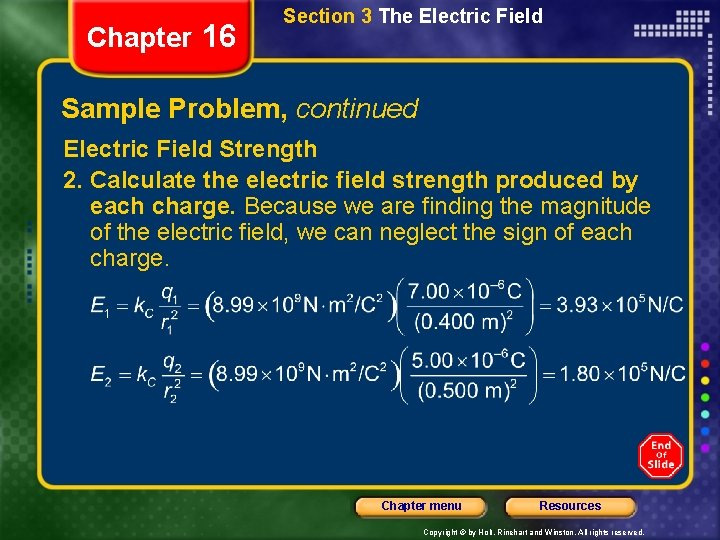Chapter 16 Section 3 The Electric Field Sample Problem, continued Electric Field Strength 2. Calculate the electric field strength produced by each charge. Because we are finding the magnitude of the electric field, we can neglect the sign of each charge. Chapter menu Resources Copyright © by Holt, Rinehart and Winston. All rights reserved.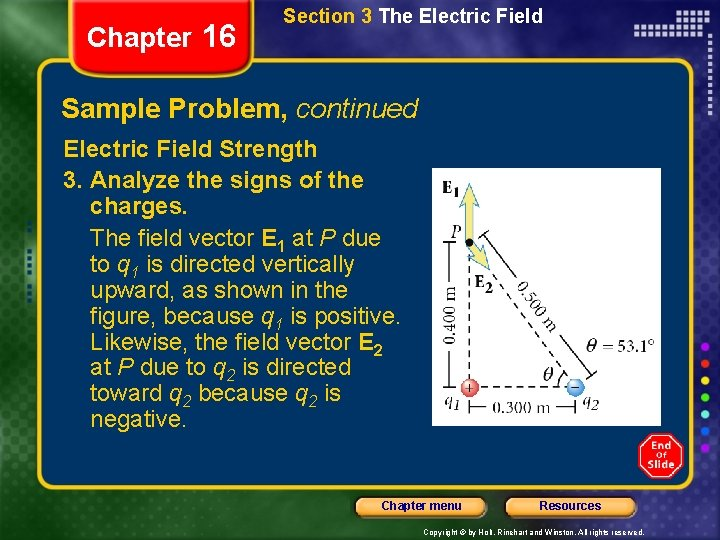Chapter 16 Section 3 The Electric Field Sample Problem, continued Electric Field Strength 3. Analyze the signs of the charges. The field vector E 1 at P due to q 1 is directed vertically upward, as shown in the figure, because q 1 is positive. Likewise, the field vector E 2 at P due to q 2 is directed toward q 2 because q 2 is negative. Chapter menu Resources Copyright © by Holt, Rinehart and Winston. All rights reserved.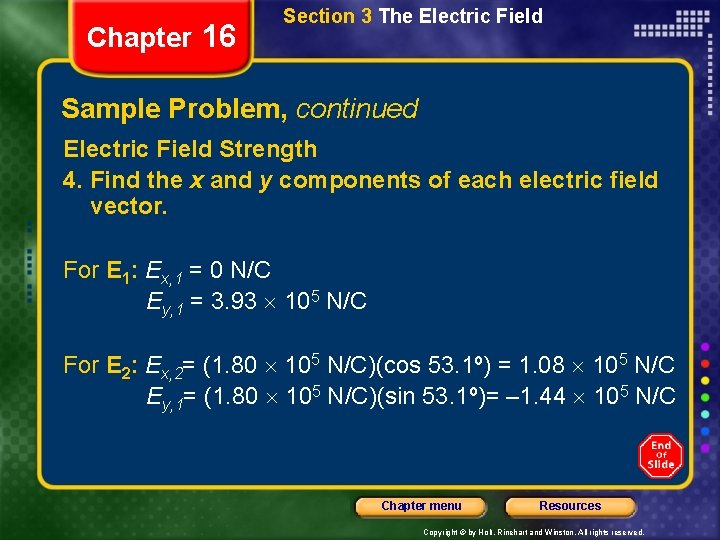Chapter 16 Section 3 The Electric Field Sample Problem, continued Electric Field Strength 4. Find the x and y components of each electric field vector. For E 1: Ex, 1 = 0 N/C Ey, 1 = 3. 93 105 N/C For E 2: Ex, 2= (1. 80 105 N/C)(cos 53. 1º) = 1. 08 105 N/C Ey, 1= (1. 80 105 N/C)(sin 53. 1º)= – 1. 44 105 N/C Chapter menu Resources Copyright © by Holt, Rinehart and Winston. All rights reserved.Chapter 16 Section 3 The Electric Field Sample Problem, continued Electric Field Strength 5. Calculate the total electric field strength in both directions. Ex, tot = Ex, 1 + Ex, 2 = 0 N/C + 1. 08 105 N/C = 1. 08 105 N/C Ey, tot = Ey, 1 + Ey, 2 = 3. 93 105 N/C – 1. 44 105 N/C = 2. 49 105 N/C Chapter menu Resources Copyright © by Holt, Rinehart and Winston. All rights reserved.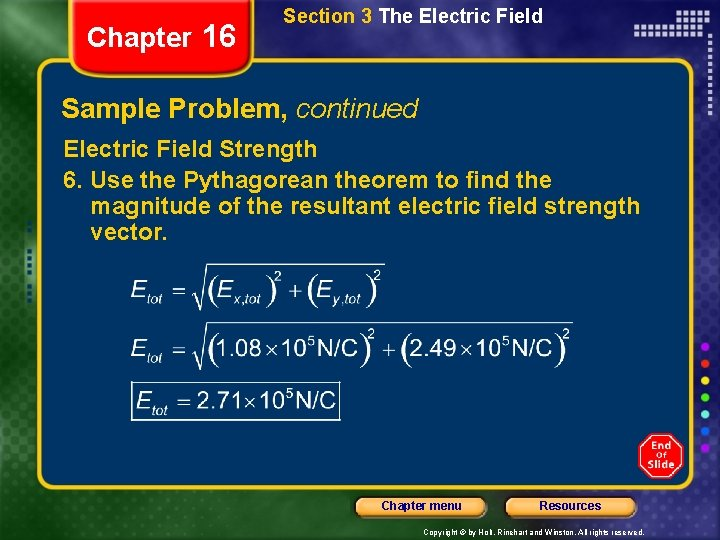Chapter 16 Section 3 The Electric Field Sample Problem, continued Electric Field Strength 6. Use the Pythagorean theorem to find the magnitude of the resultant electric field strength vector. Chapter menu Resources Copyright © by Holt, Rinehart and Winston. All rights reserved.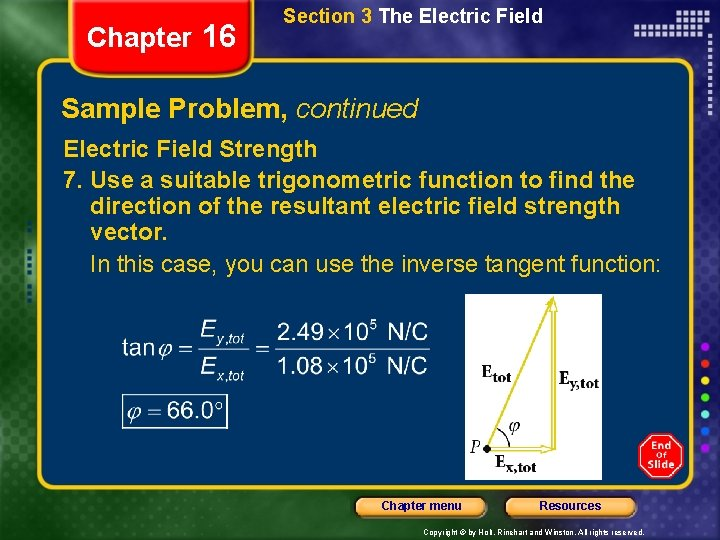Chapter 16 Section 3 The Electric Field Sample Problem, continued Electric Field Strength 7. Use a suitable trigonometric function to find the direction of the resultant electric field strength vector. In this case, you can use the inverse tangent function: Chapter menu Resources Copyright © by Holt, Rinehart and Winston. All rights reserved.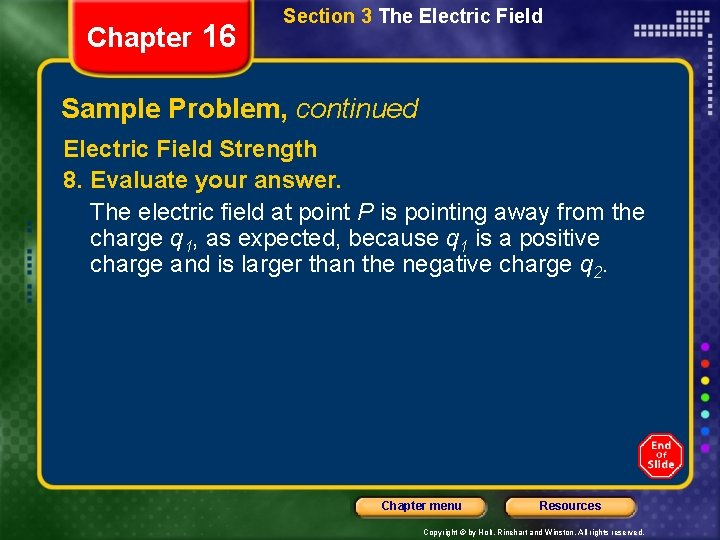Chapter 16 Section 3 The Electric Field Sample Problem, continued Electric Field Strength 8. Evaluate your answer. The electric field at point P is pointing away from the charge q 1, as expected, because q 1 is a positive charge and is larger than the negative charge q 2. Chapter menu Resources Copyright © by Holt, Rinehart and Winston. All rights reserved.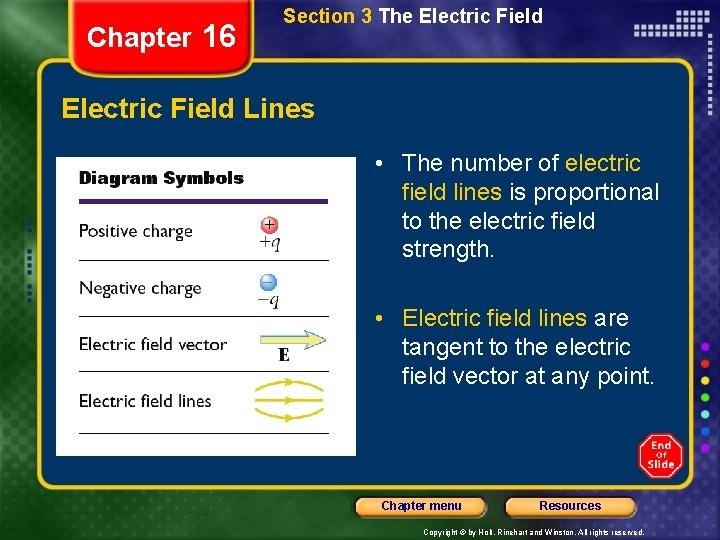Chapter 16 Section 3 The Electric Field Lines • The number of electric field lines is proportional to the electric field strength. • Electric field lines are tangent to the electric field vector at any point. Chapter menu Resources Copyright © by Holt, Rinehart and Winston. All rights reserved.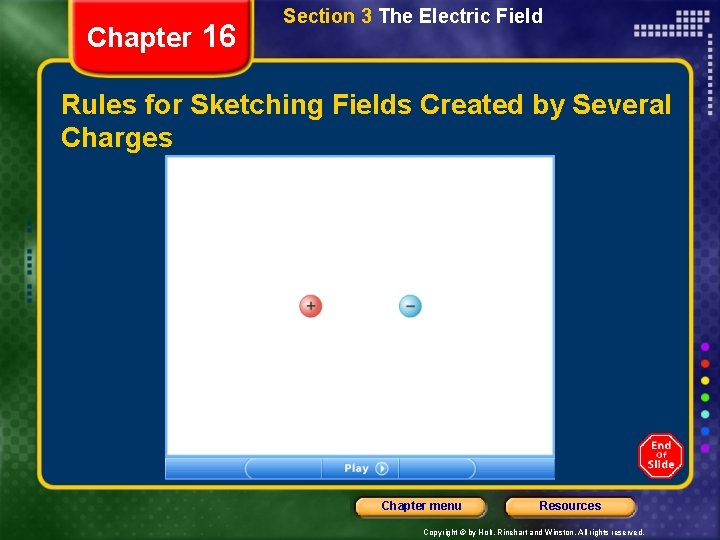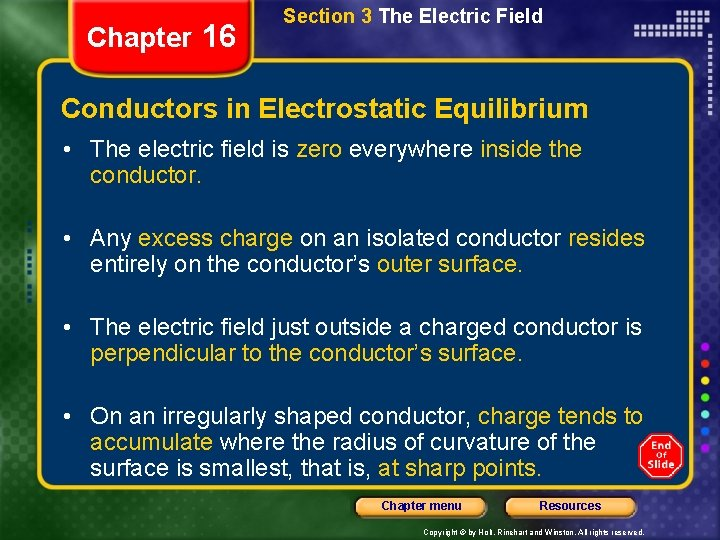Chapter 16 Section 3 The Electric Field Conductors in Electrostatic Equilibrium • The electric field is zero everywhere inside the conductor. • Any excess charge on an isolated conductor resides entirely on the conductor’s outer surface. • The electric field just outside a charged conductor is perpendicular to the conductor’s surface. • On an irregularly shaped conductor, charge tends to accumulate where the radius of curvature of the surface is smallest, that is, at sharp points. Chapter menu Resources Copyright © by Holt, Rinehart and Winston. All rights reserved.Chapter 16 Standardized Test Prep Multiple Choice 1. In which way is the electric force similar to the gravitational force? A. Electric force is proportional to the mass of the object. B. Electric force is similar in strength to gravitational force. C. Electric force is both attractive and repulsive. D. Electric force decreases in strength as the distance between the charges increases. Chapter menu Resources Copyright © by Holt, Rinehart and Winston. All rights reserved.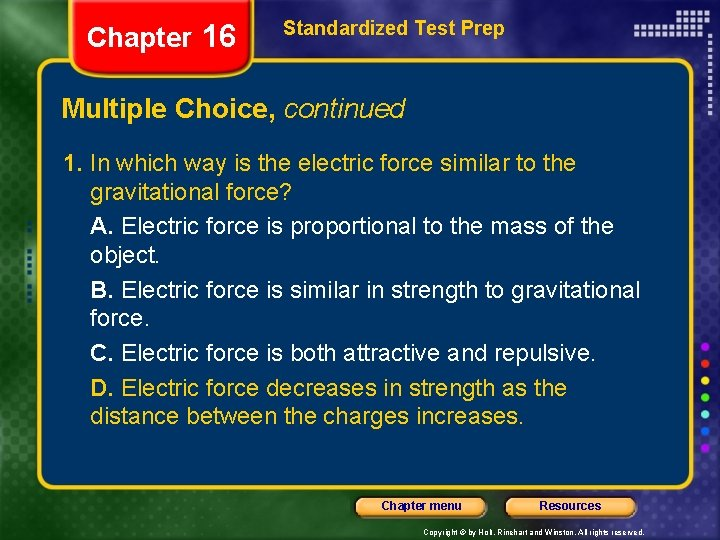Chapter 16 Standardized Test Prep Multiple Choice, continued 1. In which way is the electric force similar to the gravitational force? A. Electric force is proportional to the mass of the object. B. Electric force is similar in strength to gravitational force. C. Electric force is both attractive and repulsive. D. Electric force decreases in strength as the distance between the charges increases. Chapter menu Resources Copyright © by Holt, Rinehart and Winston. All rights reserved.Chapter 16 Standardized Test Prep Multiple Choice, continued 2. What must the charges be for A and B in the figure so that they produce the electric field lines shown? F. A and B must both be positive. G. A and B must both be negative. H. A must be negative, and B must be positive. J. A must be positive, and B must be negative. Chapter menu Resources Copyright © by Holt, Rinehart and Winston. All rights reserved.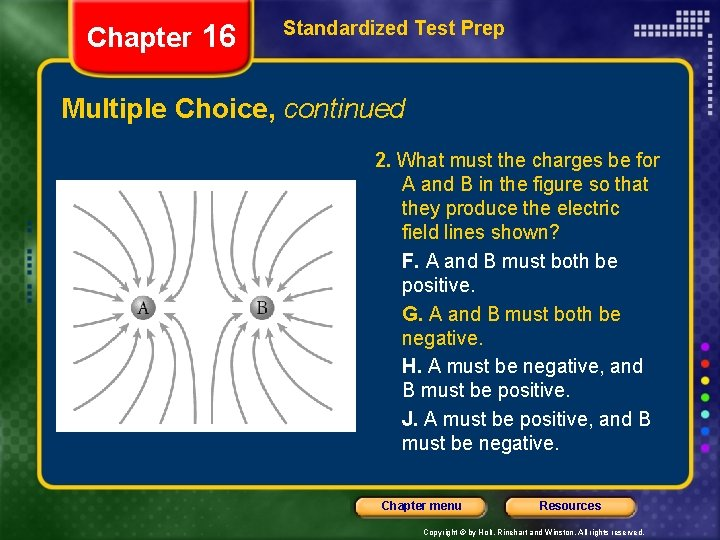Chapter 16 Standardized Test Prep Multiple Choice, continued 2. What must the charges be for A and B in the figure so that they produce the electric field lines shown? F. A and B must both be positive. G. A and B must both be negative. H. A must be negative, and B must be positive. J. A must be positive, and B must be negative. Chapter menu Resources Copyright © by Holt, Rinehart and Winston. All rights reserved.Chapter 16 Standardized Test Prep Multiple Choice, continued 3. Which activity does not produce the same results as the other three? A. sliding over a plastic-covered automobile seat B. walking across a woolen carpet C. scraping food from a metal bowl with a metal spoon D. brushing dry hair with a plastic comb Chapter menu Resources Copyright © by Holt, Rinehart and Winston. All rights reserved.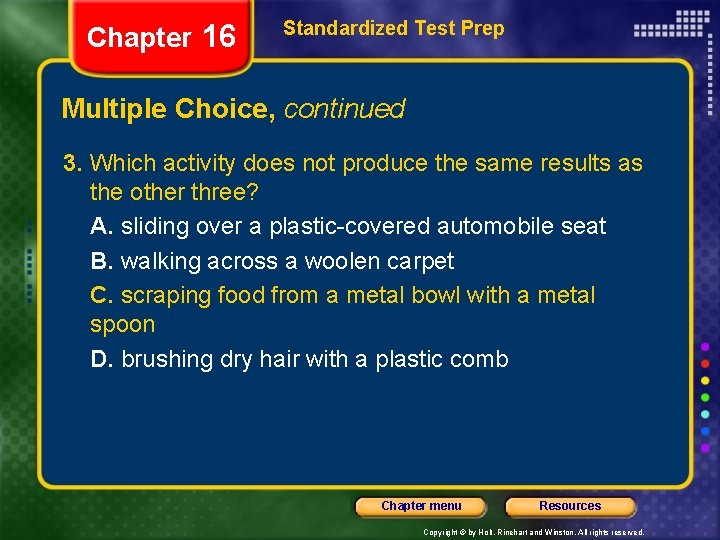Chapter 16 Standardized Test Prep Multiple Choice, continued 3. Which activity does not produce the same results as the other three? A. sliding over a plastic-covered automobile seat B. walking across a woolen carpet C. scraping food from a metal bowl with a metal spoon D. brushing dry hair with a plastic comb Chapter menu Resources Copyright © by Holt, Rinehart and Winston. All rights reserved.Chapter 16 Standardized Test Prep Multiple Choice, continued 4. By how much does the electric force between two charges change when the distance between them is doubled? Chapter menu Resources Copyright © by Holt, Rinehart and Winston. All rights reserved.Chapter 16 Standardized Test Prep Multiple Choice, continued 4. By how much does the electric force between two charges change when the distance between them is doubled? Chapter menu Resources Copyright © by Holt, Rinehart and Winston. All rights reserved.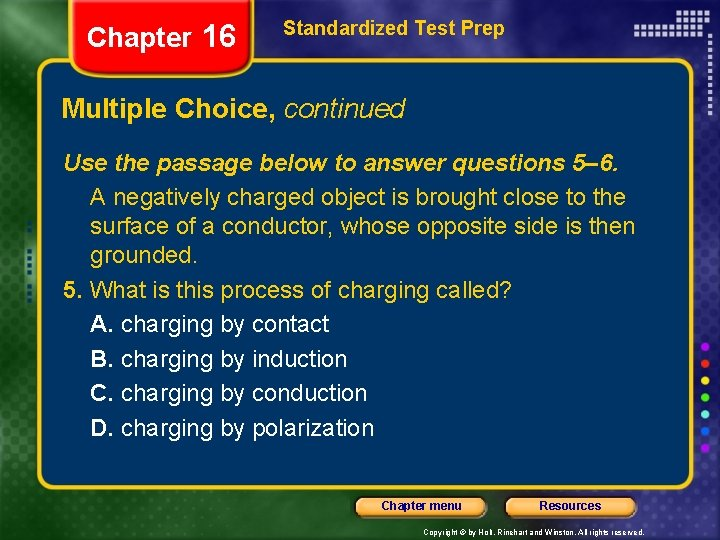Chapter 16 Standardized Test Prep Multiple Choice, continued Use the passage below to answer questions 5– 6. A negatively charged object is brought close to the surface of a conductor, whose opposite side is then grounded. 5. What is this process of charging called? A. charging by contact B. charging by induction C. charging by conduction D. charging by polarization Chapter menu Resources Copyright © by Holt, Rinehart and Winston. All rights reserved.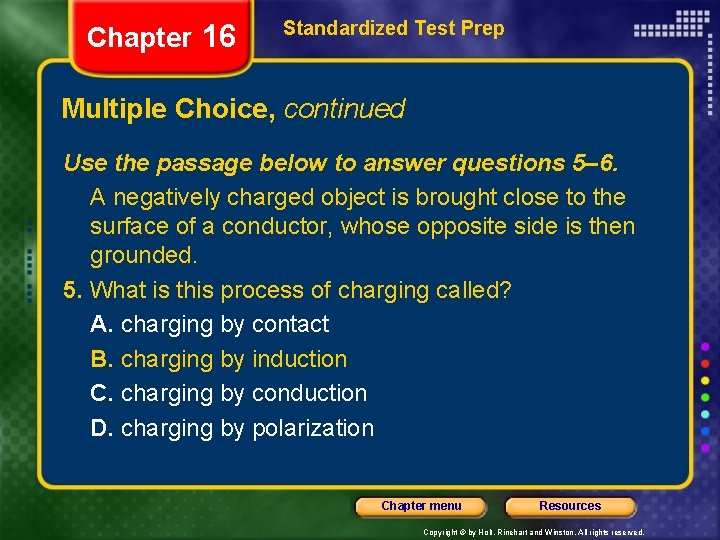Chapter 16 Standardized Test Prep Multiple Choice, continued Use the passage below to answer questions 5– 6. A negatively charged object is brought close to the surface of a conductor, whose opposite side is then grounded. 5. What is this process of charging called? A. charging by contact B. charging by induction C. charging by conduction D. charging by polarization Chapter menu Resources Copyright © by Holt, Rinehart and Winston. All rights reserved.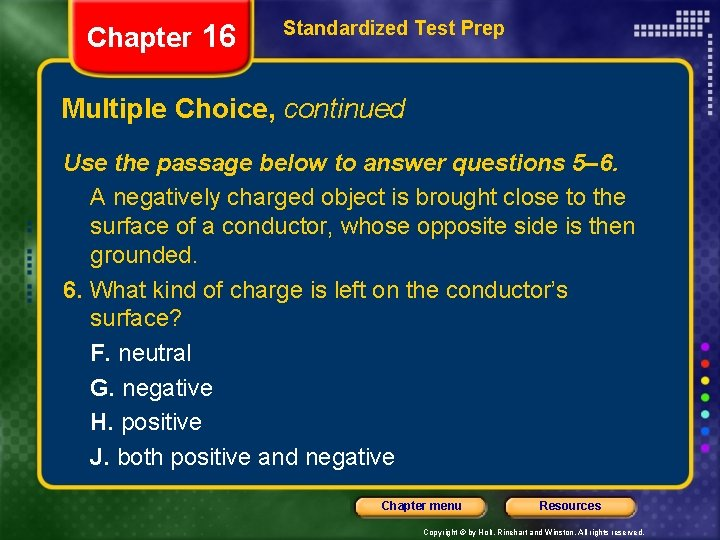Chapter 16 Standardized Test Prep Multiple Choice, continued Use the passage below to answer questions 5– 6. A negatively charged object is brought close to the surface of a conductor, whose opposite side is then grounded. 6. What kind of charge is left on the conductor’s surface? F. neutral G. negative H. positive J. both positive and negative Chapter menu Resources Copyright © by Holt, Rinehart and Winston. All rights reserved.Chapter 16 Standardized Test Prep Multiple Choice, continued Use the passage below to answer questions 5– 6. A negatively charged object is brought close to the surface of a conductor, whose opposite side is then grounded. 6. What kind of charge is left on the conductor’s surface? F. neutral G. negative H. positive J. both positive and negative Chapter menu Resources Copyright © by Holt, Rinehart and Winston. All rights reserved.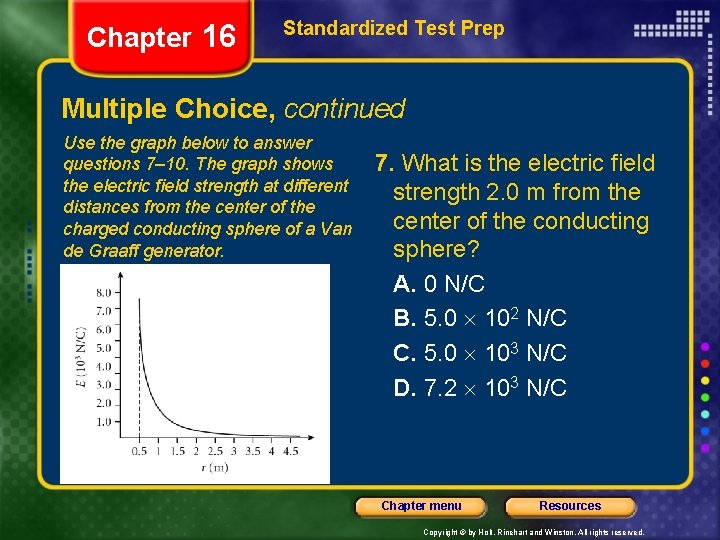Chapter 16 Standardized Test Prep Multiple Choice, continued Use the graph below to answer questions 7– 10. The graph shows the electric field strength at different distances from the center of the charged conducting sphere of a Van de Graaff generator. 7. What is the electric field strength 2. 0 m from the center of the conducting sphere? A. 0 N/C B. 5. 0 102 N/C C. 5. 0 103 N/C D. 7. 2 103 N/C Chapter menu Resources Copyright © by Holt, Rinehart and Winston. All rights reserved.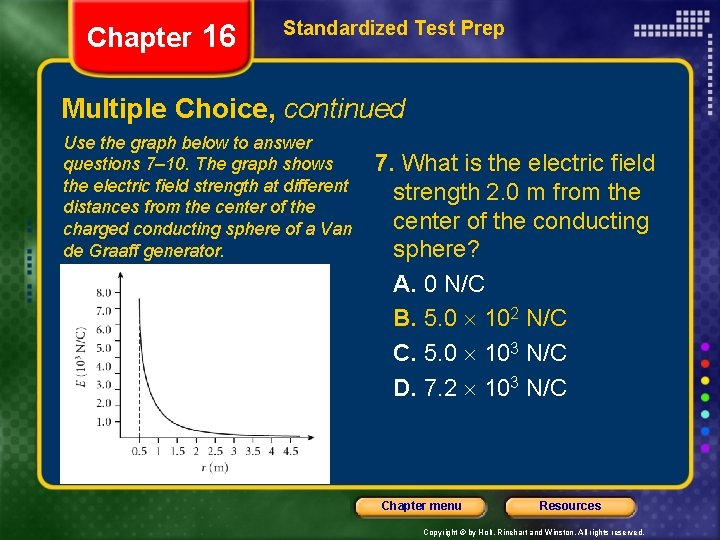Chapter 16 Standardized Test Prep Multiple Choice, continued Use the graph below to answer questions 7– 10. The graph shows the electric field strength at different distances from the center of the charged conducting sphere of a Van de Graaff generator. 7. What is the electric field strength 2. 0 m from the center of the conducting sphere? A. 0 N/C B. 5. 0 102 N/C C. 5. 0 103 N/C D. 7. 2 103 N/C Chapter menu Resources Copyright © by Holt, Rinehart and Winston. All rights reserved.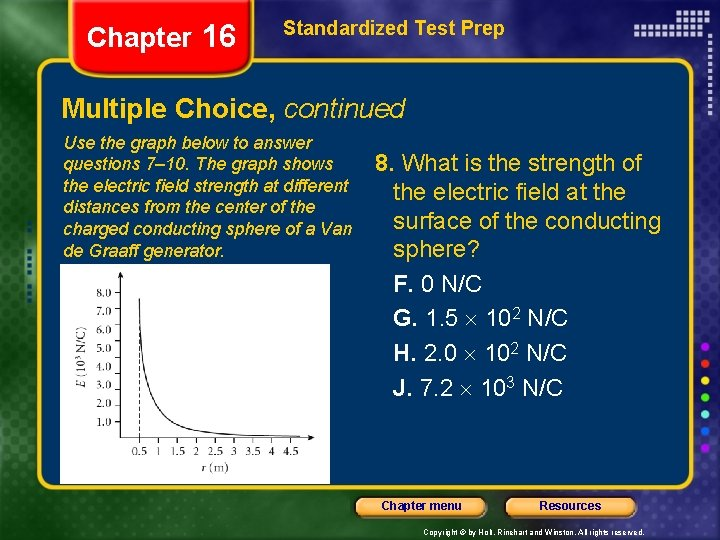Chapter 16 Standardized Test Prep Multiple Choice, continued Use the graph below to answer questions 7– 10. The graph shows the electric field strength at different distances from the center of the charged conducting sphere of a Van de Graaff generator. 8. What is the strength of the electric field at the surface of the conducting sphere? F. 0 N/C G. 1. 5 102 N/C H. 2. 0 102 N/C J. 7. 2 103 N/C Chapter menu Resources Copyright © by Holt, Rinehart and Winston. All rights reserved.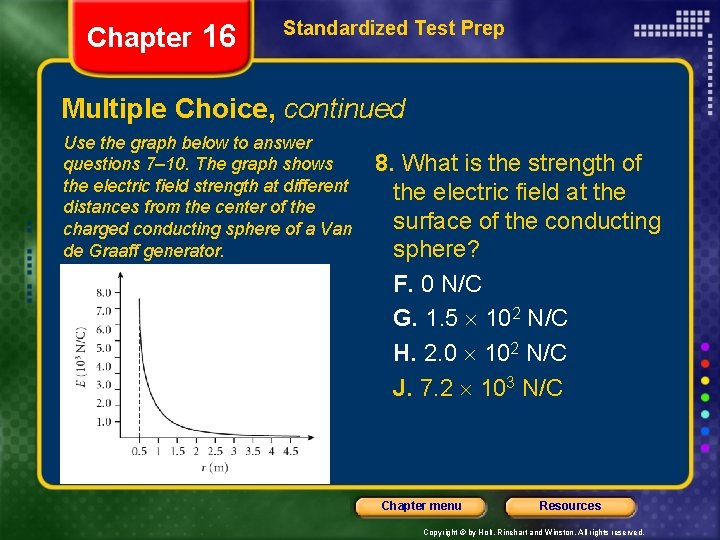Chapter 16 Standardized Test Prep Multiple Choice, continued Use the graph below to answer questions 7– 10. The graph shows the electric field strength at different distances from the center of the charged conducting sphere of a Van de Graaff generator. 8. What is the strength of the electric field at the surface of the conducting sphere? F. 0 N/C G. 1. 5 102 N/C H. 2. 0 102 N/C J. 7. 2 103 N/C Chapter menu Resources Copyright © by Holt, Rinehart and Winston. All rights reserved.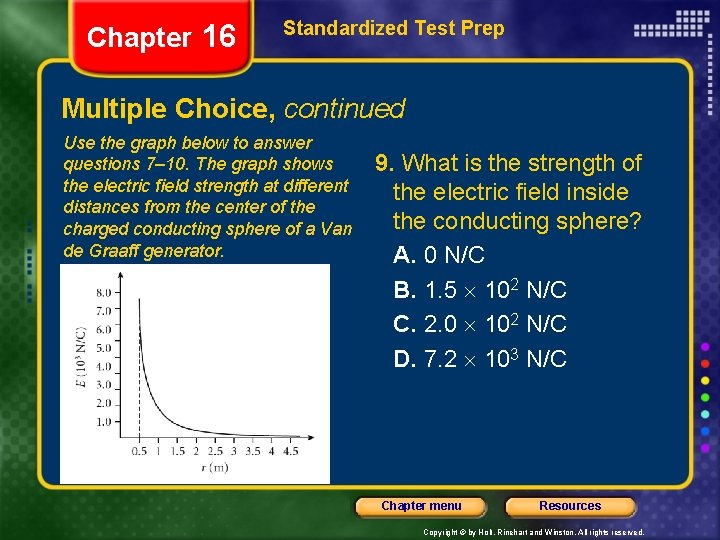Chapter 16 Standardized Test Prep Multiple Choice, continued Use the graph below to answer questions 7– 10. The graph shows the electric field strength at different distances from the center of the charged conducting sphere of a Van de Graaff generator. 9. What is the strength of the electric field inside the conducting sphere? A. 0 N/C B. 1. 5 102 N/C C. 2. 0 102 N/C D. 7. 2 103 N/C Chapter menu Resources Copyright © by Holt, Rinehart and Winston. All rights reserved.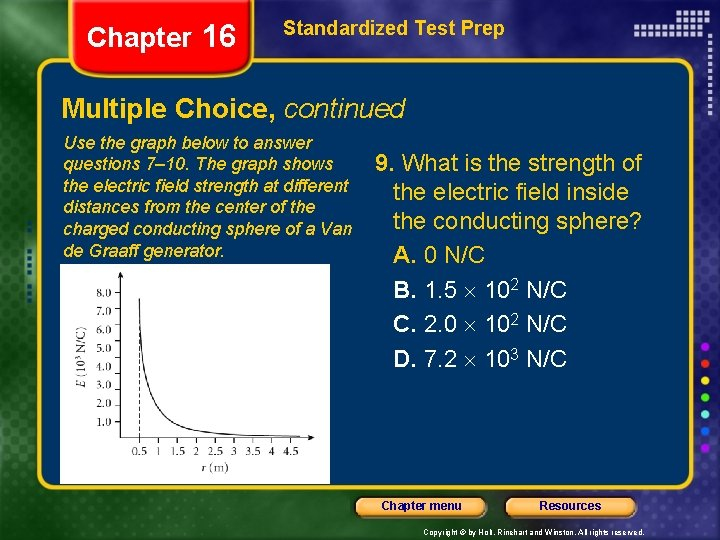Chapter 16 Standardized Test Prep Multiple Choice, continued Use the graph below to answer questions 7– 10. The graph shows the electric field strength at different distances from the center of the charged conducting sphere of a Van de Graaff generator. 9. What is the strength of the electric field inside the conducting sphere? A. 0 N/C B. 1. 5 102 N/C C. 2. 0 102 N/C D. 7. 2 103 N/C Chapter menu Resources Copyright © by Holt, Rinehart and Winston. All rights reserved.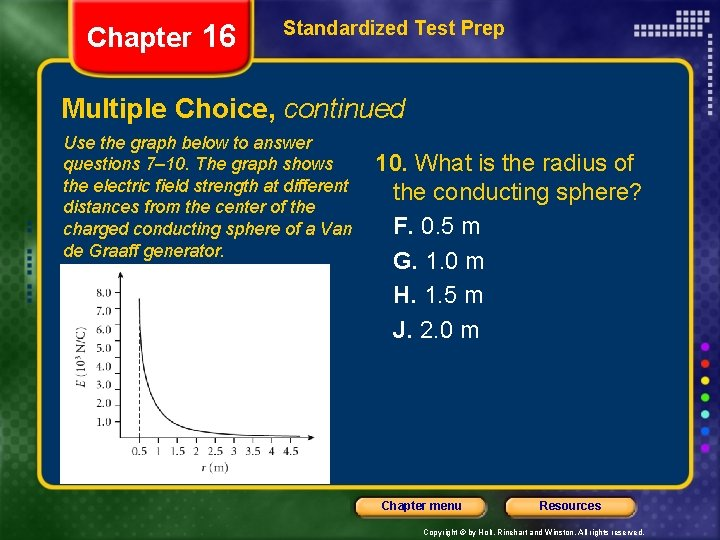Chapter 16 Standardized Test Prep Multiple Choice, continued Use the graph below to answer questions 7– 10. The graph shows the electric field strength at different distances from the center of the charged conducting sphere of a Van de Graaff generator. 10. What is the radius of the conducting sphere? F. 0. 5 m G. 1. 0 m H. 1. 5 m J. 2. 0 m Chapter menu Resources Copyright © by Holt, Rinehart and Winston. All rights reserved.Chapter 16 Standardized Test Prep Multiple Choice, continued Use the graph below to answer questions 7– 10. The graph shows the electric field strength at different distances from the center of the charged conducting sphere of a Van de Graaff generator. 10. What is the radius of the conducting sphere? F. 0. 5 m G. 1. 0 m H. 1. 5 m J. 2. 0 m Chapter menu Resources Copyright © by Holt, Rinehart and Winston. All rights reserved.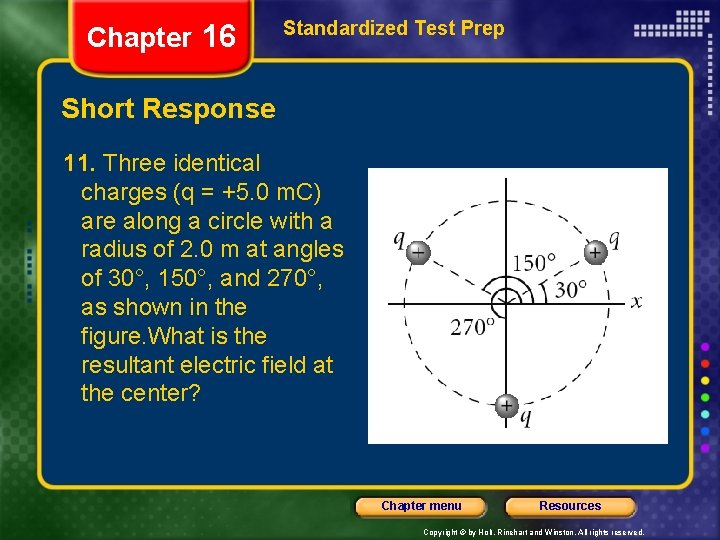Chapter 16 Standardized Test Prep Short Response 11. Three identical charges (q = +5. 0 m. C) are along a circle with a radius of 2. 0 m at angles of 30°, 150°, and 270°, as shown in the figure. What is the resultant electric field at the center? Chapter menu Resources Copyright © by Holt, Rinehart and Winston. All rights reserved.Chapter 16 Standardized Test Prep Short Response, continued 11. Three identical charges (q = +5. 0 m. C) are along a circle with a radius of 2. 0 m at angles of 30°, 150°, and 270°, as shown in the figure. What is the resultant electric field at the center? Answer: 0. 0 N/C Chapter menu Resources Copyright © by Holt, Rinehart and Winston. All rights reserved.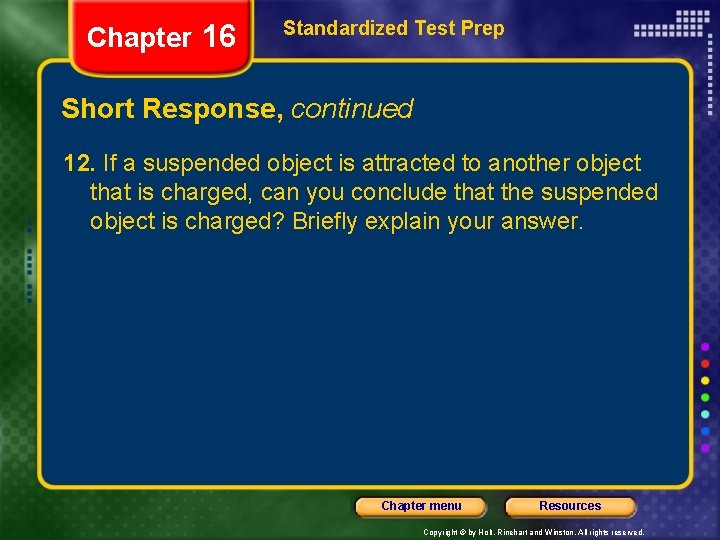Chapter 16 Standardized Test Prep Short Response, continued 12. If a suspended object is attracted to another object that is charged, can you conclude that the suspended object is charged? Briefly explain your answer. Chapter menu Resources Copyright © by Holt, Rinehart and Winston. All rights reserved.Chapter 16 Standardized Test Prep Short Response, continued 12. If a suspended object is attracted to another object that is charged, can you conclude that the suspended object is charged? Briefly explain your answer. Answer: not necessarily; The suspended object might have a charge induced on it, but its overall charge could be neutral. Chapter menu Resources Copyright © by Holt, Rinehart and Winston. All rights reserved.Chapter 16 Standardized Test Prep Short Response, continued 13. One gram of hydrogen contains 6. 02 1023 atoms, each with one electron and one proton. Suppose that 1. 00 g of hydrogen is separated into protons and electrons, that the protons are placed at Earth’s north pole, and that the electrons are placed at Earth’s south pole. Assuming the radius of Earth to be 6. 38 106 m, what is the magnitude of the resulting compressional force on Earth? Chapter menu Resources Copyright © by Holt, Rinehart and Winston. All rights reserved.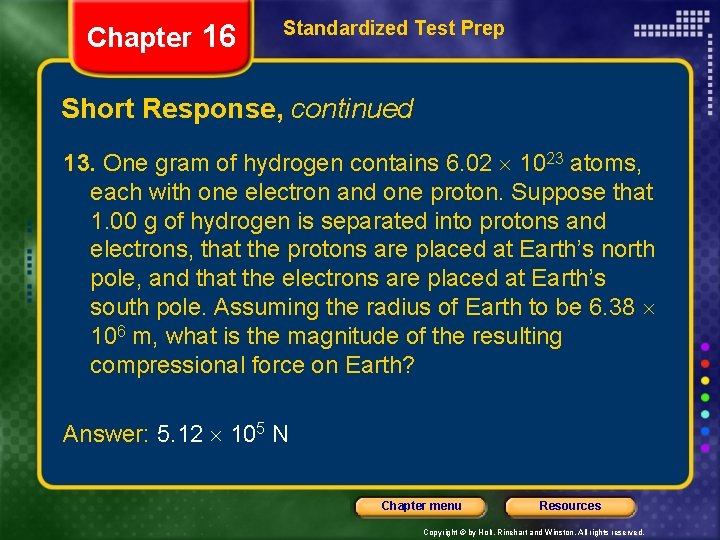Chapter 16 Standardized Test Prep Short Response, continued 13. One gram of hydrogen contains 6. 02 1023 atoms, each with one electron and one proton. Suppose that 1. 00 g of hydrogen is separated into protons and electrons, that the protons are placed at Earth’s north pole, and that the electrons are placed at Earth’s south pole. Assuming the radius of Earth to be 6. 38 106 m, what is the magnitude of the resulting compressional force on Earth? Answer: 5. 12 105 N Chapter menu Resources Copyright © by Holt, Rinehart and Winston. All rights reserved.Chapter 16 Standardized Test Prep Short Response, continued 14. Air becomes a conductor when the electric field strength exceeds 3. 0 106 N/C. Determine the maximum amount of charge that can be carried by a metal sphere 2. 0 m in radius. Chapter menu Resources Copyright © by Holt, Rinehart and Winston. All rights reserved.Chapter 16 Standardized Test Prep Short Response, continued 14. Air becomes a conductor when the electric field strength exceeds 3. 0 106 N/C. Determine the maximum amount of charge that can be carried by a metal sphere 2. 0 m in radius. Answer: 1. 3 10– 3 C Chapter menu Resources Copyright © by Holt, Rinehart and Winston. All rights reserved.Chapter 16 Standardized Test Prep Extended Response Use the information below to answer questions 15– 18. A proton, which has a mass of 1. 673 10– 27 kg, accelerates from rest in a uniform electric field of 640 N/C. At some time later, its speed is 1. 2 106 m/s. 15. What is the magnitude of the acceleration of the proton? Chapter menu Resources Copyright © by Holt, Rinehart and Winston. All rights reserved.Chapter 16 Standardized Test Prep Extended Response, continued Use the information below to answer questions 15– 18. A proton, which has a mass of 1. 673 10– 27 kg, accelerates from rest in a uniform electric field of 640 N/C. At some time later, its speed is 1. 2 106 m/s. 15. What is the magnitude of the acceleration of the proton? Answer: 6. 1 1010 m/s 2 Chapter menu Resources Copyright © by Holt, Rinehart and Winston. All rights reserved.Chapter 16 Standardized Test Prep Extended Response, continued Use the information below to answer questions 15– 18. A proton, which has a mass of 1. 673 10– 27 kg, accelerates from rest in a uniform electric field of 640 N/C. At some time later, its speed is 1. 2 106 m/s. 16. How long does it take the proton to reach this speed? Chapter menu Resources Copyright © by Holt, Rinehart and Winston. All rights reserved.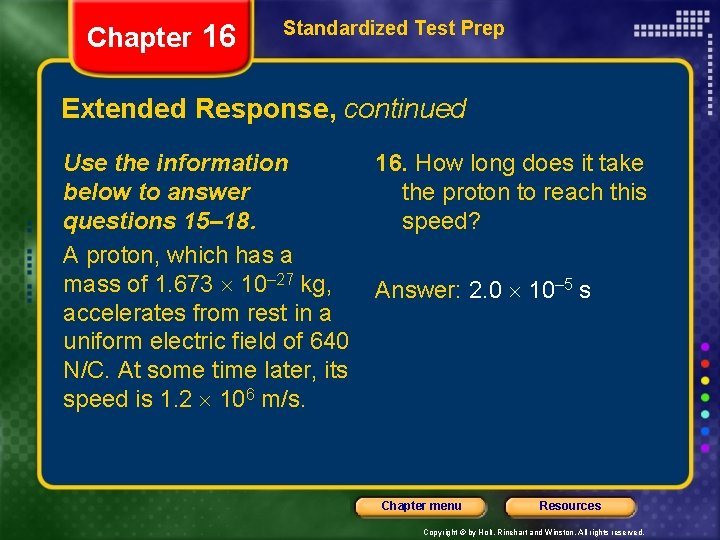Chapter 16 Standardized Test Prep Extended Response, continued Use the information below to answer questions 15– 18. A proton, which has a mass of 1. 673 10– 27 kg, accelerates from rest in a uniform electric field of 640 N/C. At some time later, its speed is 1. 2 106 m/s. 16. How long does it take the proton to reach this speed? Answer: 2. 0 10– 5 s Chapter menu Resources Copyright © by Holt, Rinehart and Winston. All rights reserved.Chapter 16 Standardized Test Prep Extended Response, continued Use the information below to answer questions 15– 18. A proton, which has a mass of 1. 673 10– 27 kg, accelerates from rest in a uniform electric field of 640 N/C. At some time later, its speed is 1. 2 106 m/s. 17. How far has it moved in this time interval? Chapter menu Resources Copyright © by Holt, Rinehart and Winston. All rights reserved.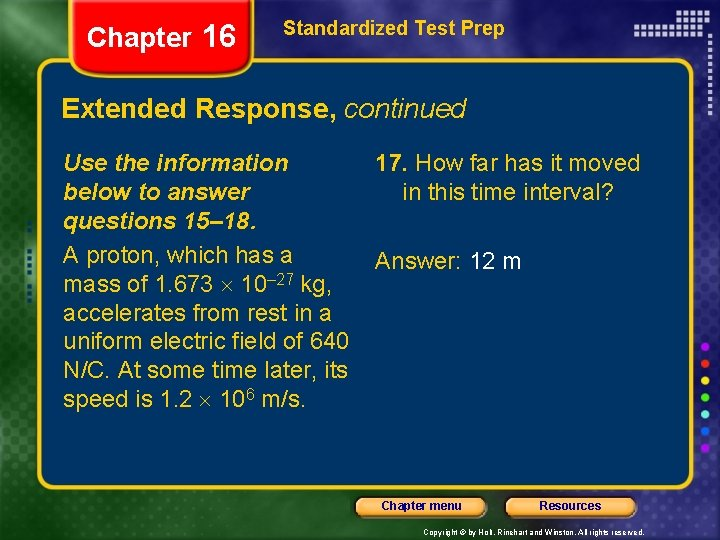Chapter 16 Standardized Test Prep Extended Response, continued Use the information below to answer questions 15– 18. A proton, which has a mass of 1. 673 10– 27 kg, accelerates from rest in a uniform electric field of 640 N/C. At some time later, its speed is 1. 2 106 m/s. 17. How far has it moved in this time interval? Answer: 12 m Chapter menu Resources Copyright © by Holt, Rinehart and Winston. All rights reserved.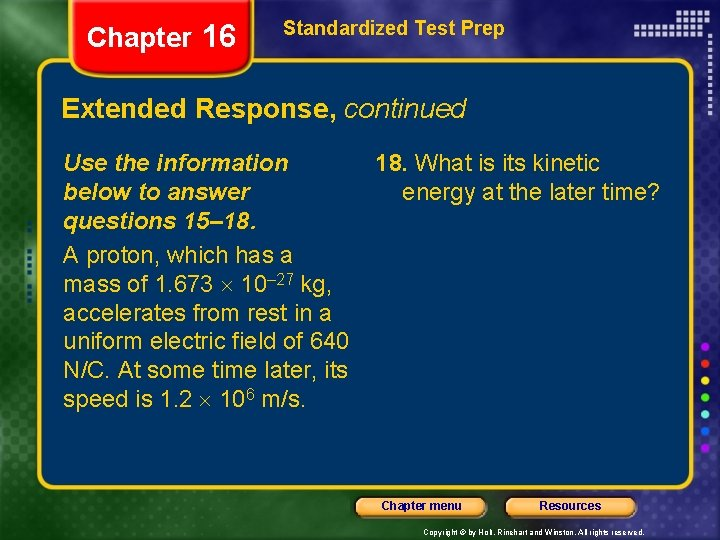Chapter 16 Standardized Test Prep Extended Response, continued Use the information below to answer questions 15– 18. A proton, which has a mass of 1. 673 10– 27 kg, accelerates from rest in a uniform electric field of 640 N/C. At some time later, its speed is 1. 2 106 m/s. 18. What is its kinetic energy at the later time? Chapter menu Resources Copyright © by Holt, Rinehart and Winston. All rights reserved.Chapter 16 Standardized Test Prep Extended Response, continued Use the information below to answer questions 15– 18. A proton, which has a mass of 1. 673 10– 27 kg, accelerates from rest in a uniform electric field of 640 N/C. At some time later, its speed is 1. 2 106 m/s. 18. What is its kinetic energy at the later time? Answer: 1. 2 10– 15 J Chapter menu Resources Copyright © by Holt, Rinehart and Winston. All rights reserved.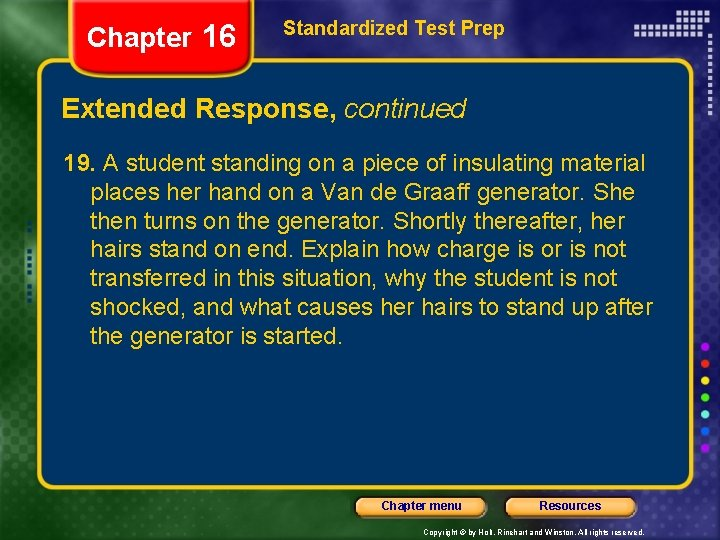Chapter 16 Standardized Test Prep Extended Response, continued 19. A student standing on a piece of insulating material places her hand on a Van de Graaff generator. She then turns on the generator. Shortly thereafter, her hairs stand on end. Explain how charge is or is not transferred in this situation, why the student is not shocked, and what causes her hairs to stand up after the generator is started. Chapter menu Resources Copyright © by Holt, Rinehart and Winston. All rights reserved.Chapter 16 Standardized Test Prep Extended Response, continued 19. (See previous slide for question. ) Answer: The charge on the sphere of the Van de Graaff generator is transferred to the student by means of conduction. This charge remains on the student because she is insulated from the ground. As there is no path between the student and the generator and the student and the ground by which charge can escape, the student is not shocked. The accumulation of charges of the same sign on the strands of the student’s hair causes the strands to repel each other and so stand on end. Chapter menu Resources Copyright © by Holt, Rinehart and Winston. All rights reserved.